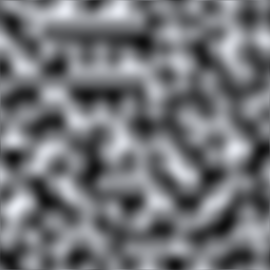﻿ Unity - スクリプティング API: Mathf.PerlinNoise

• C#
• JS
• Boo

## スクリプト言語

お好みのスクリプト言語を選択すると、サンプルコードがその言語で表示されます。

# Mathf.PerlinNoise

Suggest a change

## Success!

Thank you for helping us improve the quality of Unity Documentation. Although we cannot accept all submissions, we do read each suggested change from our users and will make updates where applicable.

Close

## Sumbission failed

For some reason your suggested change could not be submitted. Please try again in a few minutes. And thank you for taking the time to help us improve the quality of Unity Documentation.

Close

Cancel

public static function PerlinNoise(x: float, y: float): float;
public static float PerlinNoise(float x, float y);
public static def PerlinNoise(x as float, y as float) as float

## Returns

float Value between 0.0 and 1.0.

## Description

2D のパーリンノイズを生成します

Perlin noise is a pseudo-random pattern of float values generated across a 2D plane (although the technique does generalise to three or more dimensions, this is not implemented in Unity). The noise does not contain a completely random value at each point but rather consists of "waves" whose values gradually increase and decrease across the pattern. The noise can be used as the basis for texture effects but also for animation, generating terrain heightmaps and many other things.Perlin noise sampled in the range 0..10 (the greyscale values represent values from 0..1) Any point in the plane can be sampled by passing the appropriate X and Y coordinates. The same coordinates will always return the same sample value but the plane is essentially infinite so it is easy to avoid repetition by choosing a random area to sample from.

```	// Create a texture and fill it with Perlin noise.
// Try varying the xOrg, yOrg and scale values in the inspector
// while in Play mode to see the effect they have on the noise.

// Width and height of the texture in pixels.
var pixWidth: int;
var pixHeight: int;

// The origin of the sampled area in the plane.
var xOrg: float;
var yOrg: float;

// The number of cycles of the basic noise pattern that are repeated
// over the width and height of the texture.
var scale = 1.0;

private var noiseTex: Texture2D;
private var pix: Color[];

function Start () {
// Set up the texture and a Color array to hold pixels during processing.
noiseTex = new Texture2D(pixWidth, pixHeight);
pix = new Color[noiseTex.width * noiseTex.height];
renderer.material.mainTexture = noiseTex;
}

function CalcNoise() {
// For each pixel in the texture...
for (var y = 0.0; y < noiseTex.height; y++) {
for (var x = 0.0; x < noiseTex.width; x++) {
// Get a sample from the corresponding position in the noise plane
// and create a greyscale pixel from it.
var xCoord = xOrg + x / noiseTex.width * scale;
var yCoord = yOrg + y / noiseTex.height * scale;
var sample = Mathf.PerlinNoise(xCoord, yCoord);
pix[y * noiseTex.width + x] = new Color(sample, sample, sample);
}
}

// Copy the pixel data to the texture and load it into the GPU.
noiseTex.SetPixels(pix);
noiseTex.Apply();
}

function Update () {
CalcNoise();
}
```
```using UnityEngine;
using System.Collections;

public class ExampleClass : MonoBehaviour {
public int pixWidth;
public int pixHeight;
public float xOrg;
public float yOrg;
public float scale = 1.0F;
private Texture2D noiseTex;
private Color[] pix;
void Start() {
noiseTex = new Texture2D(pixWidth, pixHeight);
pix = new Color[noiseTex.width * noiseTex.height];
renderer.material.mainTexture = noiseTex;
}
void CalcNoise() {
float y = 0.0F;
while (y < noiseTex.height) {
float x = 0.0F;
while (x < noiseTex.width) {
float xCoord = xOrg + x / noiseTex.width * scale;
float yCoord = yOrg + y / noiseTex.height * scale;
float sample = Mathf.PerlinNoise(xCoord, yCoord);
pix[y * noiseTex.width + x] = new Color(sample, sample, sample);
x++;
}
y++;
}
noiseTex.SetPixels(pix);
noiseTex.Apply();
}
void Update() {
CalcNoise();
}
}
```
```import UnityEngine
import System.Collections

public class ExampleClass(MonoBehaviour):

public pixWidth as int

public pixHeight as int

public xOrg as float

public yOrg as float

public scale as float = 1.0F

private noiseTex as Texture2D

private pix as (Color)

def Start() as void:
noiseTex = Texture2D(pixWidth, pixHeight)
pix = array[of Color]((noiseTex.width * noiseTex.height))
renderer.material.mainTexture = noiseTex

def CalcNoise() as void:
y as float = 0.0F
while y < noiseTex.height:
x as float = 0.0F
while x < noiseTex.width:
xCoord as float = (xOrg + ((x / noiseTex.width) * scale))
yCoord as float = (yOrg + ((y / noiseTex.height) * scale))
sample as float = Mathf.PerlinNoise(xCoord, yCoord)
pix[((y * noiseTex.width) + x)] = Color(sample, sample, sample)
x++
y++
noiseTex.SetPixels(pix)
noiseTex.Apply()

def Update() as void:
CalcNoise()

```

Although the noise plane is two-dimensional, it is easy to use just a single one-dimensional line through the pattern, say for animation effects.

```	// "Bobbing" animation from 1D Perlin noise.

// Range over which height varies.
var heightScale = 1.0;

// Distance covered per second along X axis of Perlin plane.
var xScale = 1.0;

function Update () {
var height = heightScale * Mathf.PerlinNoise(Time.time * xScale, 0.0);
var pos = transform.position;
pos.y = height;
transform.position = pos;
}
```
```using UnityEngine;
using System.Collections;

public class ExampleClass : MonoBehaviour {
public float heightScale = 1.0F;
public float xScale = 1.0F;
void Update() {
float height = heightScale * Mathf.PerlinNoise(Time.time * xScale, 0.0F);
Vector3 pos = transform.position;
pos.y = height;
transform.position = pos;
}
}
```
```import UnityEngine
import System.Collections

public class ExampleClass(MonoBehaviour):

public heightScale as float = 1.0F

public xScale as float = 1.0F

def Update() as void:
height as float = (heightScale * Mathf.PerlinNoise((Time.time * xScale), 0.0F))
pos as Vector3 = transform.position
pos.y = height
transform.position = pos

```